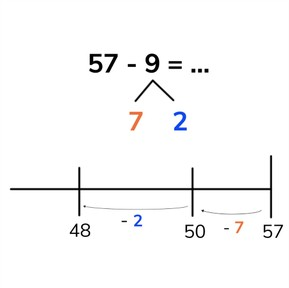Subtraction to 100 by splitting the subtrahend with subtrahends < 10

# Subtraction to 100 by splitting the subtrahend with subtrahends < 108,000 schools use Gynzy92,000 teachers use Gynzy1,600,000 students use Gynzy

## General

Students learn to subtract numbers to 100 and use the strategy to split the subtrahend with jumps on the number line when the subtrahend is less than 10.

2.NBT.B.5

## Relevance

Discuss with students that they can subtract by splitting the second number into smaller parts with problems in which it is difficult to subtract the second number all at once. An example is if you want to know how many kids are at a club training if there are 92 kids in the club and 8 are not present.

## Introduction

Practice splitting numbers less than 10 into two smaller numbers. Students will need to know how to do this to cross tens.

## Development

Explain to students that when splitting the subtrahend, you take the subtrahend away from the minuend (first number) in two steps. You split the subtrahend into two smaller numbers and subtract those smaller numbers from the minuend. Explain to students that this can be helpful for crossing tens. Show students what this looks like on the number line. Show students a subtraction problem and place the minuend at the end of the number line and ask students to think about what the nearest tens number is. Have students split the subtrahend into two numbers, one that is required to jump to the previous tens number, and the rest which they jump after. Ask students where they end up. This number is your difference. Then practice splitting the subtrahend again where only the jumps are shown on the number line, and finally practice with a blank number line.

Check that students are able to subtract by splitting the subtrahends with subtrahends less than 10 by asking the following question:

## Guided practice

Student first are given a subtraction problem in which the jumps are shown on the number line. They are then given problems in which they must jump on the number line, and finally must do all the jumps on the number line. The problems all have visual support.

## Closing

Discuss with students that it is important to be able to subtract numbers to 100 by splitting the subtrahends and subtracting on the number line so you can easily subtract numbers. Show the number line on the interactive whiteboard and ask students which subtraction problem is shown. Then have them solve the subtraction problem by drawing the jumps on the number line. Erase the grey boxes to check the answers. Discuss all the jumps shown on the number lines.

## Teaching tips

Students who have difficulty splitting the subtrahends can make use of a number line. Have them solve the problems by taking away the subtrahend in jumps on the number line. Emphasize that they first need to jump to the nearest tens number and then take away the rest.

### The online teaching platform for interactive whiteboards and displays in schools

• Save time building lessons

• Manage the classroom more efficiently

• Increase student engagement